## General Chemistry

We mentioned in the previous post that covalent bonds are formed as a result of sharing two valence electrons in overlapping orbitals of two atoms.

For example, the following Lewis structures represent covalent bonds together with some lone pairs of electrons:Now, there are two types of covalent bonds: sigma (σ) and pi (π) bonds. The bonds that form by the head-on overlap of orbitals are called σ (sigma) bonds because the electron density is concentrated on the axis connecting the two atoms. In short, you can remember that single bonds are sigma bonds. For example, the single bond between the two carbons in ethane (C2H6) is a sigma bond because it is formed by overlapping two sp3 orbitals of the adjacent carbon atoms: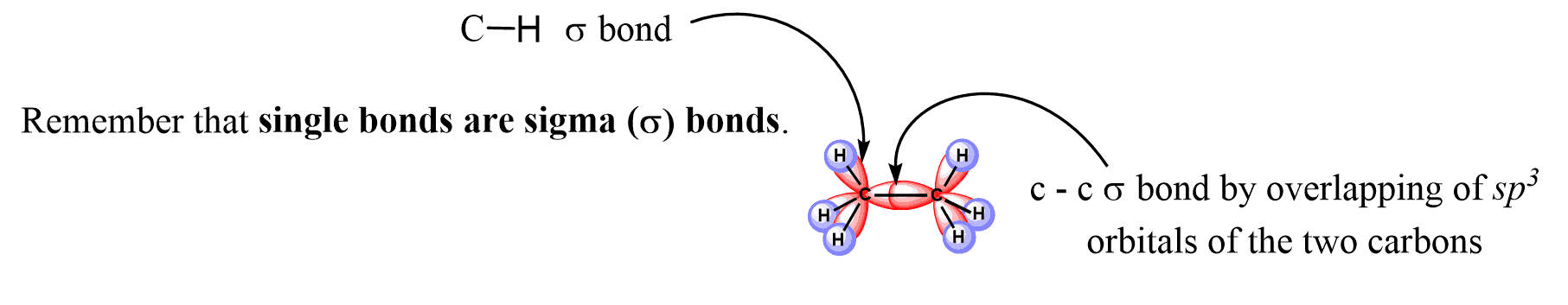On the other hand, in ethene, or ethylene (C2H4), there is one sigma and one pi bond between the two carbon atoms:

The pi bond is formed by a side-to-side overlap of two p orbitals provided by adjacent atoms.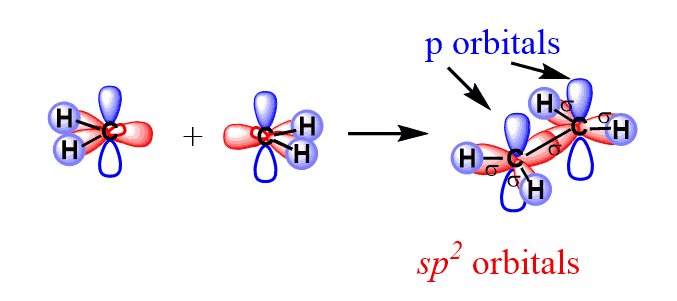The two carbons are sp2-hybridized where the sp2 orbitals overlap to form the sigma bond, and the unhybridized p orbitals form the pi bond. Together they make the C=C double bond.

So, the two carbons in ethylene, which is the first member of the alkene family, are double-bonded. In each double bond, there is one sigma and one π bond.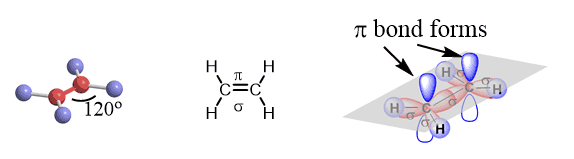Here are some key parameters about the sp2 hybridization and double bonds that you need to know:

* All the atoms on the double bond are in one plane.

* The angle between atoms is 120o.

* The angle between the plane and p orbitals is 90o.

The side-to-side orbital overlap allows a less efficient electron sharing, and therefore, p bonds are generally weaker than sigma bonds.  This is why in organic chemistry, you will see reactions involving breaking pi bonds far more often than sigma bonds as they are broken much easier.

An interesting feature distinguishing sigma and pi bonds is the former is characterized by free rotation about the bond axis, while the presence of a pi bond restricts this, and the atoms are locked in their position relative to the double bond. This results in what is called the cis and trans isomerism in alkenes which, again, we will talk about in organic chemistry.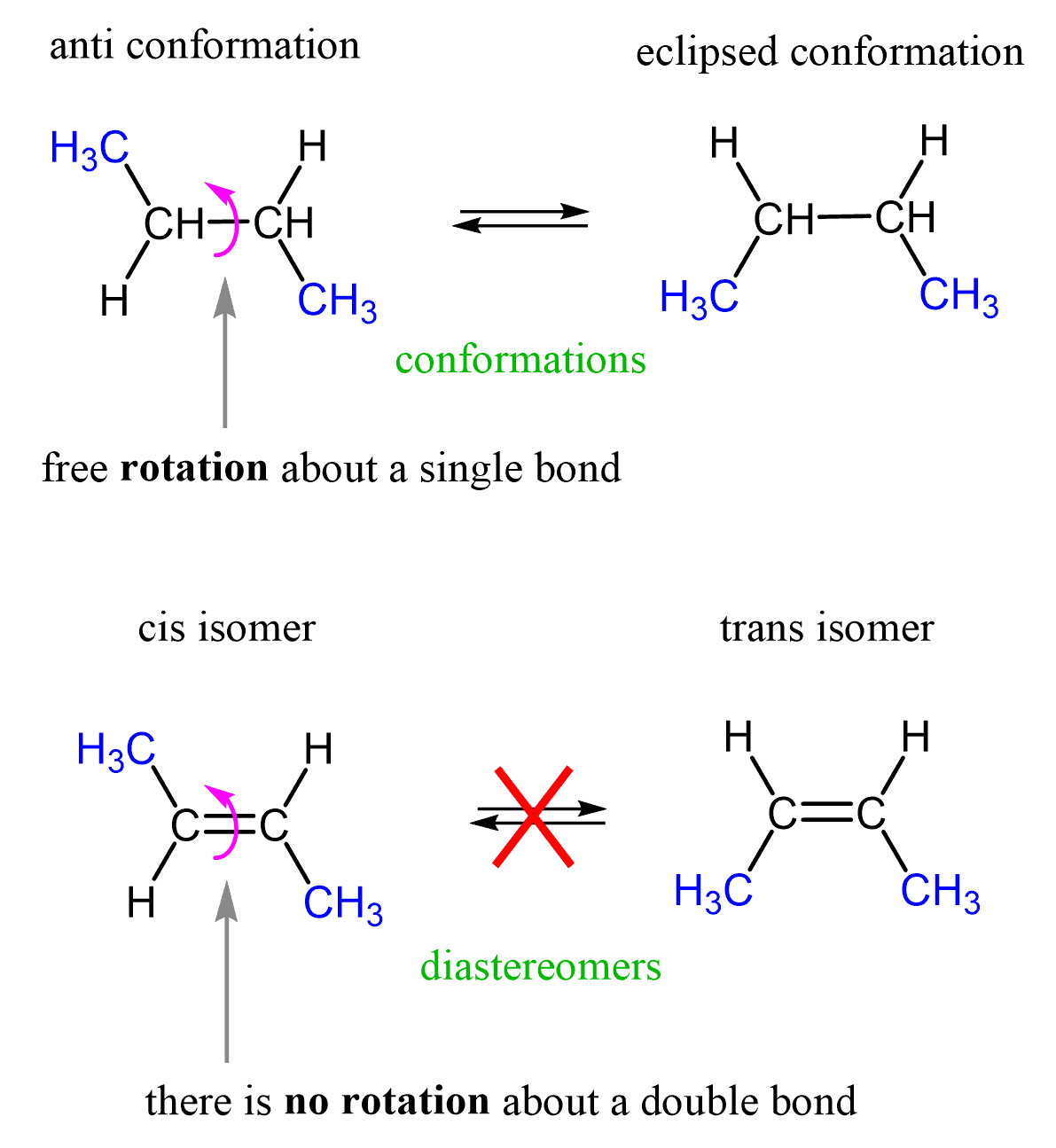As a shortcut to recognizing the sigma and pi bonds, you can remember that in multiple bonds (double or triple), there is always one sigma bond, and the remaining are pi bonds. For example, in acetylene, C2H2, the carbons are connected with a triple bond in which one is a sigma bond formed by the head-on overlap of the two sp orbitals and two pi bonds which are formed by overlapping two pairs of p orbitals: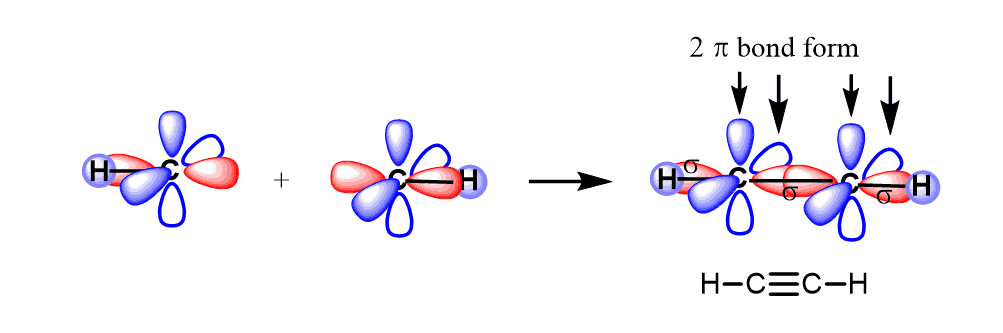One hydrogen bonds to each carbon atom by overlapping its s orbital with the other sp orbital.

The two p orbitals of each carbon overlap to make two π bonds.

The key parameters of the sp hybridization and triple bond:

* All the atoms have linear geometry.

* The angle between atoms is 180o linear geometry.

* In a triple bond there is one σ (sigma) and two π (pi) bonds.

# More Examples of Sigma and Pi Bonds

The principle of identifying sigma and pi bonds is applicable to any molecule, be that smaller or large than what we have discussed so far. For example, in carbon dioxide, CO2, each single between the carbon and oxygens is a sigma bond, and there is a pi bond making two C=O double bonds:Similar to this, the triple bond between the nitrogen atoms in N2 gas is made of one sigma and two pi bonds.Check Also